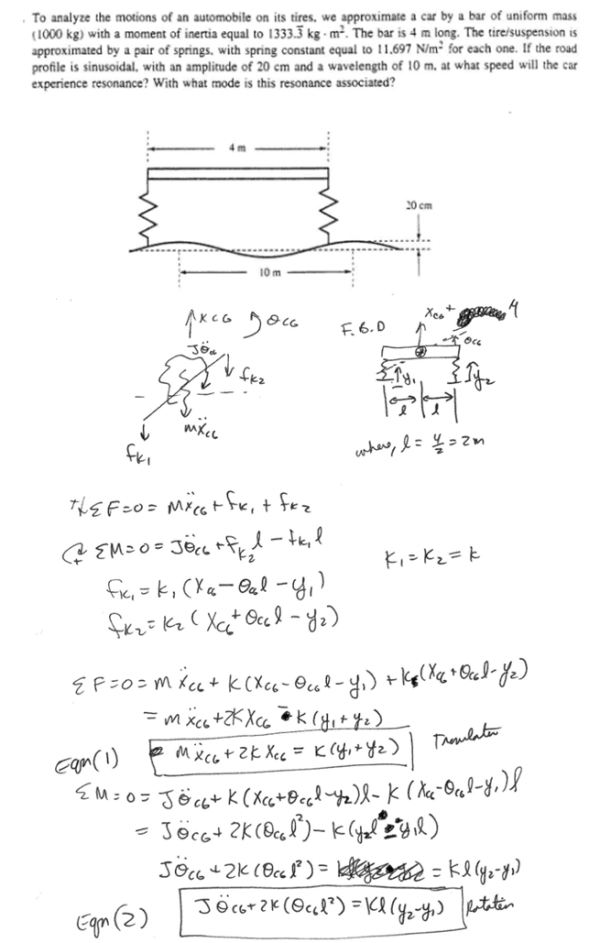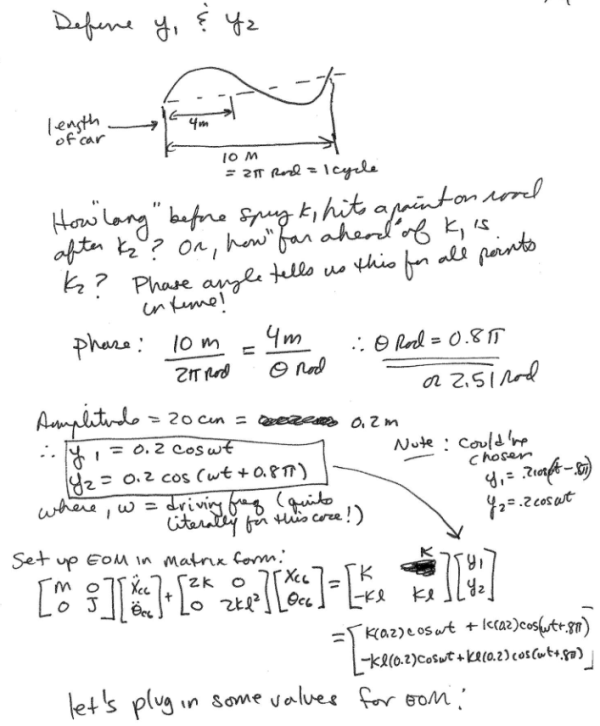To analyze the motions of an automobile on its tires, we approximate a car by a bar of uniform mass (1000 kg) with a moment of inertia equal to 1333.3 kg m^2. The bar is 4 m long. The tire/suspension is approximated by a pair of springs with spring constant equal to 11.697 N/m^2 for each one. If the road profile is sinusoidal, with an amplitude of 20 cm and a wavelength of 10 m, at what speed will the car experience resonance? with what mode is this resonance associated?To analyze the motions of an automobile on its tires, we approximate a car by a bar of uniform mass (1000 kg) with a moment of inertia equal to 1333.3 kg m^2. The bar is 4 m long. The tire/suspension is approximated by a pair of springs with spring constant equal to 11.697 N/m^2 for each one. If the road profile is sinusoidal, with an amplitude of 20 cm and a wavelength of 10 m, at what speed will the car experience resonance? with what mode is this resonance associated?

Vibrations Page 1 vibrations vibrations vibrations vibrations vibrations vibrations vibrations Vibrations Page 2